When a data set contains a large number of repeated values, you can simplify the process of computing the mean by using weights — the frequencies of a value in a sample or a population. You can then compute the geometric mean as a weighted average.

You can calculate the weighted geometric mean in the same way for both samples and populations. The formula is: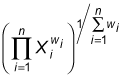Here's the breakdown of this equation: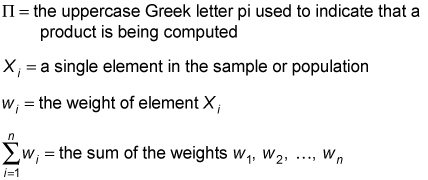You apply an exponent to each element in the data set that equals the weight of the element. You then multiply these values together and raise to a power equal to one divided by the sum of the weights.

An exponent is the superscript in an expression such as 34; in this case, the base is 3 and the exponent is 4. This is shorthand for multiplying 3 by itself four times: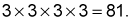Note that in many formulas and Microsoft Excel, the asterisk (*) represents multiplication. In Excel the carat (^) represents exponentiation.

As an example, a marketing firm conducts a survey of 20 households to determine the average number of cellphones each household owns. Here's the sample data from this survey:

Number of Cell Phones Per Household Number of Households
1 2
2 5
3 6
4 4
5 3

To figure out the weighted geometric mean, follow these steps:

1. Compute the value of each Xi with an exponent equal to its weight wi: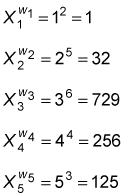2. Multiply these results together: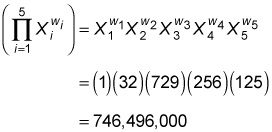3. Divide 1 by the sum of the weights: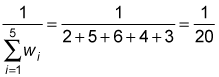4. Combine these results to find the weighted geometric mean: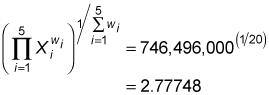So on average, each household has approximately 2.78 cellphones.